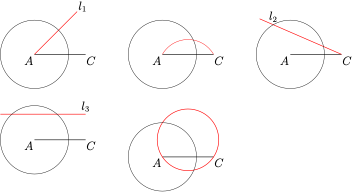# 每日一题构图

A．$\cos A$

B．$\cos B$

C．$\cos C$

D．$\triangle ABC$ 的面积

E．$\triangle ABC$ 的外接圆半径